# Some Solid (Three-dimensional) Geometrical Facts about the Golden Section

Having looked at the flat geometry (two dimensional) of the number Phi, we now find Phi in the most symmetrical of the three-dimensional solids - the Platonic Solids.
The calculators on this page require JavaScript but you appear to have switched JavaScript off (it is disabled). Please go to the Preferences for this browser and enable it if you want to use the calculators.
The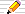icon means there is a You do the maths... section of questions to start your own investigations. The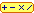calculator icon indicates that there is a live interactive calculator in that section.

1·61803 39887 49894 84820 45868 34365 63811 77203 09179 80576 ..More..## Phi and 3-dimensional geometry

The five regular solids (where "regular" means all sides are equal and all angles are the same and all the faces are identical) are called the five Platonic solids after the Greek philosopher and mathematician, Plato. Euclid also wrote about them. For more information on these two famous Greeks, see the footnote.

## Dice shapes

What shapes make the best dice?
We need to make sure all the faces are the same shape and that all the angles and sides are equal, or some faces will be favoured more than others and so our dice will be "unfair".
The dice you usually find today are cube-shaped - 6 square faces, all angles are right-angles and all sides are the same length.

[There are other shapes that make fair dice if we relax these conditions a little. Can you guess what they are? See the footnote for the answers.]
There are only FIVE fair-dice-shapes altogether if we strictly insist on the following conditions:

all sides are equal in length and
all angles are equal so that
all the faces are identical in shape and size

## Coordinates and other statistics of the 5 Platonic Solids

They are the tetrahedron, cube (or hexahedron), octahedron, dodecahedron and icosahedron.

Their names come from the number of faces (hedron=face in Greek and its plural is hedra). tetra=4, hexa=6, octa=8, dodeca=12 and icosa=20.

Remember that in these pages Phi is 1·61803.. and phi is 1/Phi = Phi-1 = 0·61803... .

The Solid images can be rotated ( press the button) as can the Stereo views. For the auto-stereographic views, either cross your eyes or keep your eyes focussed in the distance until the two images fuse into one and you see the shape in depth. If you place your mouse on the "rotate" button before you do this then a quick click will make it appear to rotate in 3-dimensions.
You may notice a pause when first seeing a rotating diagram while it is being downloaded.

The "wire-frame" views are symmetrical plan views of the frame of the object with wire edges and the faces missing, dotted lines being edges that would be hidden by solid faces.

### The Tetrahedron

 Solid View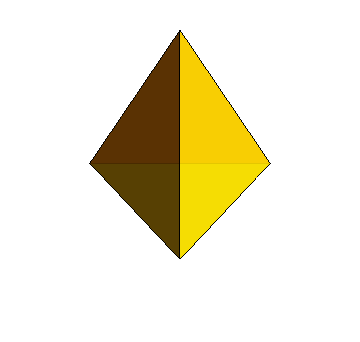Stereo View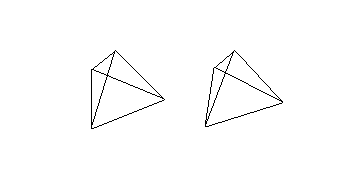Wireframe Views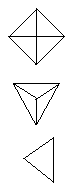4 vertices with coordinates: (1, 1, 1), (1, -1, -1), (-1, 1, -1), (-1, -1, 1) 6 edges of length 2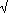2, mid point of edge to centre of solid = 1 4 triangular faces, Surface area = 8 √3, volume = 8/3,

### The Cube or Hexahedron

 Solid View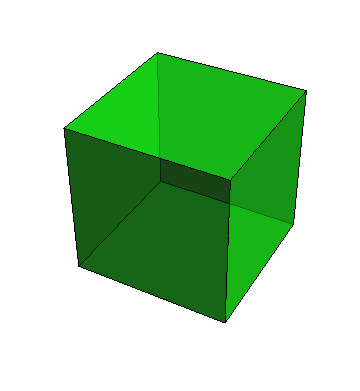Stereo View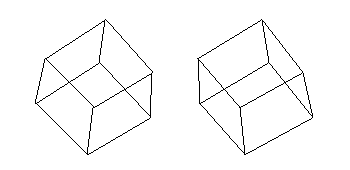Wireframe Views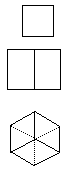8 vertices with coordinates (±1, ±1, ±1), i.e.: (1, 1, 1), (1, 1, -1), (1, -1, 1), (1, -1, -1), (-1, 1, 1), (-1, 1, -1), (-1, -1, 1), (-1, -1, -1) 12 sides of length 2, mid point of edge to centre of solid = √2 6 square faces each of area 4, surface area = 24, volume 8

### The Octahedron

 Solid View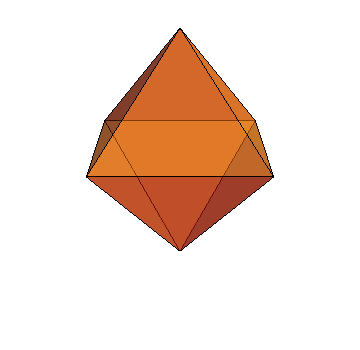Stereo View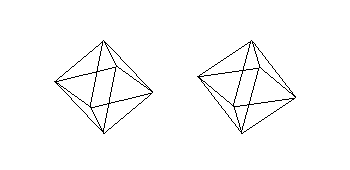Wireframe Views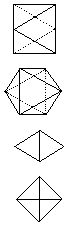6 vertices with coordinates (±1, 0 0), (0, ±1, 0), (0, 0, ±1) i.e.: (1, 0, 0), (-1, 0, 0), (0, 1, 0), (0, -1, 0), (0, 0, 1), (0, 0, -1) 12 sides of length2, mid point of edge to centre of solid = 1/2 8 triangular faces, surface area = 4√3, volume 4/3

### The Dodecahedron

 Solid View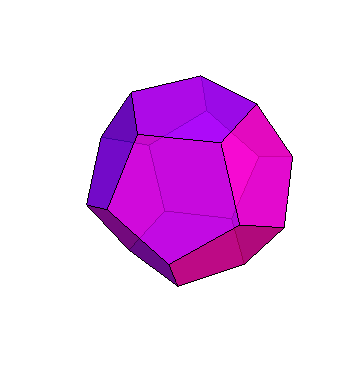Stereo View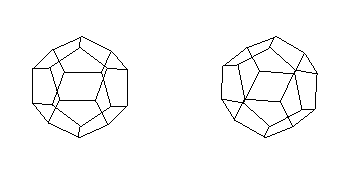Wireframe Views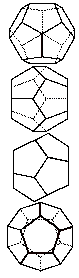20 vertices with coordinates (0, ±phi, ±Phi),(±Phi, 0, ±phi),(±phi, ±Phi, 0), (±1, ±1, ±1), i.e.: (0, phi, Phi), (0, phi, -Phi), (0, -phi, Phi), (0, -phi, -Phi), (Phi, 0, phi), (Phi, 0, -phi), (-Phi, 0, phi), (-Phi, 0, -phi), (phi, Phi, 0), (phi, -Phi, 0), (-phi, Phi, 0), (-phi, -Phi, 0), (1, 1, 1), (1, 1, -1), (1, -1, 1), (1, -1, -1), (-1, 1, 1), (-1, 1, -1), (-1, -1, 1), (-1, -1, -1) 30 sides of length 2 phi, mid point of edge to centre of solid = Phi, vertex to centre =3 =(phi2 + Phi2) 12 pentagonal faces, surface area = 12 √5 phi = √360(5 − √5) = 15 √5 Phi + 10 , volume = 8 + 4 Phi = 10 + 25

### The Icosahedron

 Solid View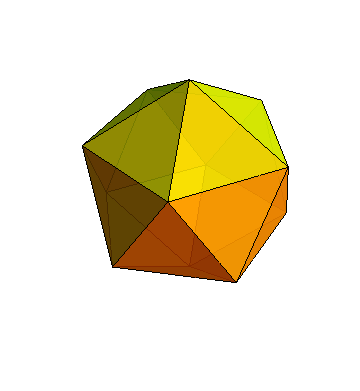Stereo View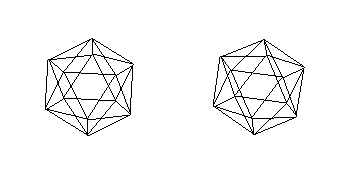Wireframe Views12 vertices with coordinates (0, ±Phi, ±1), (±1, 0, ±1Phi), (±Phi, ±1, 0) i.e.: (0, Phi, 1), (0, Phi, -1), (0, -Phi, 1), (0, -Phi, -1), (1, 0, Phi), (1, 0, -Phi), (-1, 0, Phi), (-1, 0, -Phi), (Phi, 1, 0), (Phi, -1, 0), (-Phi, 1, 0), (-Phi, -1, 0) 30 sides of length 2, mid point of edge to centre of solid = (1+Phi)/3 20 triangular faces, Surface area = 203, volume = 20( 1 + Phi)/3 = 20 Phi2/3 = 10 (3 +5)/3

## The Dual of a Solid

There are two more important relationships between the dodecahedron and the icosahedron. First, the mid-points of the faces of the dodecahedron define the points on an icosahedron and the mid-points of the faces of an icosahedron define a dodecahedron. The same is true of the cube and the octahedron. If we try it with a tetrahedron, we just get another tetrahedron. Each is called the dual of the other solid where the number of edges in each pair is the same, but the number of faces of one is the number of points of the other, and vice-versa.

### Golden sections in the Dodecahedron, Icosahedron and Octahedron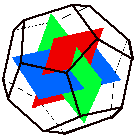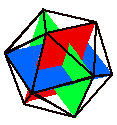If we join mid-points of the dodecahedron's faces, we can get three rectangles all at right angles to each other. What's more, they are Golden Rectangles since their edges are in the ratio 1 to Phi.
The same happens if we join the vertices of the icosahedron since it is the dual of the dodecahedron.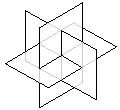Using these golden rectangles it is easy to see that the coordinates of the icosahedron are as given above since they are:
(0,± 1, ± Phi), (± Phi, 0, ± 1), (± 1, ± Phi, 0) .

####You do the maths...1. Here is an interesting way to make a model of an icosahedron based on the three golden rectangles intersecting as in the picture above:
• Cut out three golden rectangles. One way to do this is to take three postcards or other thin card and cut them so they are 10cm by 16.2cm.
• In the centre of each, make a cut parallel to the longest side which is as long as the shortest side of a card.
The three cards will be slotted through these slits to make the arrangement in the picture above. To do this, on one of the cards extend the cut to one of the edges.
```     +--------------+ Make  and one   +-------------+
!              ! two       of    !             !
!    ======    ! of        these !   ===========
!              ! these           !             !
+--------------+                 +-------------+
```
• Assemble the cards so that they look like the picture here of the red, green and blue rectangles. [This is a nice little puzzle itself!] You may want to put pieces of sticky-tape where two cards meet just to make it a bit more stable.
• Now you can make an icosahedron by joining the corners of the rectangles by glueing cotton so that it looks like the picture above.
• It will be quite delicate, so tape another piece of cotton to one of the short edges of one of the cards and hang it up like a mobile!
2. If you are good at coordinate geometry or like a challenge, then show that the 12 points of the icosahedron divide the edges of the octahedron in the ratio Phi:1 (or 1:phi if you like) where the octahedron has vertices at:
( ±Phi2 , 0 0 ), ( 0, ±Phi2 , 0 ), ( 0, 0, ±Phi2 )
[from H S M Coxeter's book Introduction to Geometry, 1961, page 163.]

### A Cube in a Dodecahedron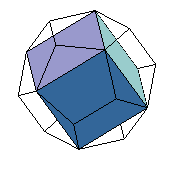We can see a cube in a dodecahedron if we use one diagonal on each face. Since the diagonals of a dodecahedron are Phi times as long as the sides (see Pentagons and Pentagrams on the Phi and 2D Geometry page at this site), then cube's sides and the dodecahedron's sides are in the golden ratio.
With thanks to Prof Susan Goldstine.
In fact, 5 distinct cubes can be fitted into the dodecahedron, with the vertices of the cubes meeting at the vertices of the dodecahedron as shown in the pictures below.
Notice that:
1. The cubes are red, green, yellow, blue and magenta.
2. Each of the 5 cubes has 12 edges, totalling 60 in the dodecahedron and so each of the 12 faces of the dodecahedron will have 5 of the edges in it.
In fact, each face of the dodecahedron will have a pentagram on it formed from just one of the edges of each of the 5 cubes.
3. No edges will overlap in any of the cubes but each cube edge will cross the one edge of 2 of the other cubes.
4. Each of the 5 cubes has 8 vertices making 40 cube-corners to share among the 20 vertices of the dodecahedron. Each vertex of the dodecahedron is shared with exactly 2 of the 5 cubes.

 Click on these buttons to see the individual and combined solids.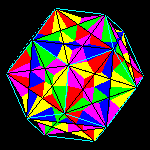The animation is 284K so you may notice a small downloading delay when you click on the "Rotate.." button.

### An Icosahedron in an Octahedron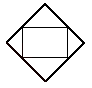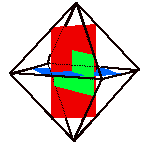Using the same three golden rectangles at right-angles to each other, we can also make an octahedron.
If we put a square as shown around each rectangle, the squares will also be at right angles to each other and form the edges of an octahedron.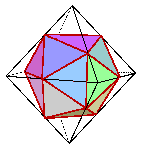Now if we join the "golden-section points" forming the corners of our three rectangles (and now on both the edges of an octahedron and also forming the vertices of an icosahedron as we saw above), we can see how to fit an icosahedron into an octahedron - and the process involves golden sections!

Here are some more Platonic-solids-within-Platonic-solids: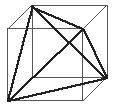A Tetrahedron in a Cube
Select one corner of a cube and join it to the opposite corner on each face.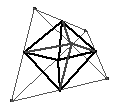An Octahedron in a Tetrahedron
Join the mid-point of each edge to any other edge mid-point where the connecting line lies on one face of the tetrahedron.An Octahedron in a Cube
Join the mid-points of faces: if two faces are next to each other at a corner, then their mid-points can be joined.

## The Greeks, Kepler and the Five ElementsThe Greeks saw great significance in the existence of just 5 Platonic solids and they related them to the 4 ELEMENTS (fire, earth, air and water) that they thought everything was made from. Together with the UNIVERSE, they associated each with a particular solid.
The astronomer and mathematician, Kepler (1571-1630), shown here as a link to the History of Mathematics web site at St Andrews University, Scotland, justified this as follows:
Of the 5 solids, the tetrahedron has the smallest volume for its surface area and the icosahedron the largest; they therefore show the properties of dryness and wetness respectively and so correspond to FIRE and WATER.
The cube, standing firmly on its base, corresponds to the stable EARTH but the octahedron which rotates freely when held by two opposite vertices, corresponds to the mobile AIR.
The dodecahedron corresponds to the UNIVERSE because the zodiac has 12 signs (the constellations of stars that the sun passes through in the course of one year) corresponding to the 12 faces of the dodecahedron.
Kepler called the golden section "the division of a line into extreme and mean ratio", as did the Greeks. He wrote the following about it:
"Geometry has two great treasures: one is the Theorem of Pythagoras; the other, the division of a line into extreme and mean ratio. The first we may compare to a measure of gold; the second we may name a precious jewel."
Johannes Kepler, (1571-1630)
• Raoul Martens recommends an article in German on Kepler's interest in the Platonic solids: Die kosmische Funktion des Goldenen Schnitts by Theodor Landscheidt in Sterne, Mond, Kometen, Bremen und die Astronomie zum 75. Jahrestag der Olbers-Gesell-schaft Bremen e.V. Verlag H. M. Hauschild, Bremen 1995.

1·61803 39887 49894 84820 45868 34365 63811 77203 09179 80576 ..More..## Quasicrystals and Phi

On the Flat Phi page, we saw that the two triangles that appear in the pentagram and pentagon were used by Roger Penrose to design tiling patterns with five-fold symmetry called Penronse tilings. Is there a three-dimensional analogue of those two-dimensional tilings? The answer, thought to be impossible until Penrose's work of the early 1970's showed that there could be structures that filled space (in the same way that tilings fill planes) that have five-fold symmetry.

### Are any Platonic solids space-filling?

#### The Cube

Yes, since identical copies of a cube can be stacked to fill a volume of space as large as we like with no gaps. Any child playing with cubical bricks learns how to build a wall or a bigger cube from the little ones, and the process can be continued indefinitely to fill all space.

#### Tetrahedrons and Octahedrons

The tetrahedron and the octahedron used together will fill space with no gaps since one fills the gaps left by the other. But neither the tetrahedron on its own nor the octahedron on its own will pack space without leaving gaps.
To see this, look at the "Tetrahedron In A Cube" picture above. We can imagine a kind of 3-D "graph paper" filled with cubes and in one cube we will place a tetrahedron. It uses just 4 of the 8 vertices of its cube, so at each of these vertices in all the cubes at those vertices, we place more tetrahedra. We repeat on the surrounding cubes and in so doing, we split all the vertices into two sets - those on a tetrahedron and those not on one. Each vertex not on a tetrahedron is the centre of one octahedron. To see this visit Mark Somer's web pages of Amy C. Edmondson's book A Fuller Explanation about the geometry of R Buckminster Fuller, chapter 12 Figure 12.2 for a helpful diagram. [The online book is worth browsing through as it has lots more interesting geometry about space filling shapes.]

#### Icosahedrons, Dodecahedrons

Similarly, neither will the icosahedron nor the dodecahedron, as it is analogous to trying to tile a plane with pentagons - they leave odd gaps that are not pentagonal and both the dodecahedron and the icosahedron exhibit five-fold symmetry too. To see this, look back at the sections above on the Icosahedron and Dodecahedron and you will find that, in the "other views" each has a view with five-fold symmetry. These views correspond to looking along an axis through the centre of the solids which have five-fold symmetry.

### Quasicrystals

Penrose found that there are two simple shapes that you can use to fill a flat space as large as you like and that have five-fold axes of symmetry. The shapes are built from 6 flat faces which are rhombuses, that is, shapes with all sides of equal length (like a square) and which has opposite sides parallel (again like a square), but which does not have all its angles equal - so they are diamond shaped (rhombs, rhombuses). The Penrose tiling shown on the Flat Phi page is also made from two rhombuses and fills the plane with a five-fold symmetric pattern.
For the solid shapes, the faces are all diamonds (rhombs) but not the ones used in the Penrose tiling and pentagons and pentagrams.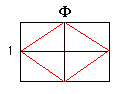The surprising relationship that holds for these new rhombuses is that
the ratio of the two diagonals of the diamonds (rhombuses) is Phi!
So this is a different rhomb from the Penrose rhombs and we shall call it the golden rhomb.
This makes the semi-angles (half the angles inside the rhombus) have tangents of Phi and phi so the angles of the rhombus are 2x31·717474..° = 2x0·55357435889r and 2x58·282525588° = 2x1·0172219674r.
[The angles in the rhombs in the Penrose tiling are 2/5 pi and 3/5 pi (72° and 108°) in one and 1/5 pi and 4/5 pi (36° and 144°) in the other.]

The two solids are similar to a cube but the faces are golden rhombs. The first shape is made by attaching three golden rhombs at their shorter angles in the same way as three squares meet at a corner of a cube. A duplicate is made and the two fit together to make a six-sided shape like a slanted cube. This is called a prolate rhombohedron.
The other shape is made by joining three golden rhombs together in the same way but at the larger angles this time. A duplicate of this is again fitted to make a different six-sided cube-like shape. This is called an oblate rhombohedron.
The two shapes look like cubes leaning over to one side.
Take a large number of one of these shapes and you can indeed fill as large a space as you like with them. When stacking cubes or octahedra, all the shapes are aligned identically (look identical, with the same orientation). When we use a rhombohedron, some must be turned round to fit in with others. These also occur in nature, although only discovered since the 1950's and, because they are not quite as symmetrical as crystals, as called quasi-crystals.

### Do quasicrystals occur in nature too?

Yes they do and a large number of substances have now been identified with such structures.

Crystals, the most symmetrical structures (with identical orientation for all the building blocks) are seen in sugar and salt as well as diamonds and quartz. Quasicrystals are an unsuspected new state of matter, sharing some of the properties of crystals and also on non-crystalline matter (such as glass). In 1984 the "impossible" five-fold symmetry was observed in an aluminium-manganese alloy (Al6Mn) and the term quasicrystal was invented for it in: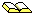D Shechtman, I Blech, D Gratias, J W Cahn Metallic phase with long-range orientational order and no translational symmetry Physics Review Letters 1984, Vol 53, pages 1951-1953.

• H S M Coxeter's Regular Polytopes, (Third Ed) 1973, Dover, is a very popular book at an amazingly low price - well worth getting!
• H S M Coxeter, Introduction to Geometry, 1961, John Wiley, is a classic! See especially section 11.2: De Divina Proportione.
• The classic and encyclopaedic book on tilings is Grunbaum and Shepard's Tilings and Patterns W H Freeman and Co, 1989. It is well worth dipping into just to admire the pictures and patterns as the maths in it is sometimes beyond school level i.e. post age 16. Nevertheless, it is an inspiring book and chock full of interesting results on all kinds of patterns and tilings with copious illustrations. There is also a cheaper paperback version but both forms are now out of print. If you can pick up a second-hand copy, it's well worth the effort if you want to study tilings seriously.
• Polyominoes: A Guide to Puzzles and Problems in tiling George Martin, the Mathematical Association of America (1991), 184 pages, is about those wonderful polyominoes puzzles. A polyomino is an extension of a domino of which is just 1 shape that we can make from two squares.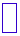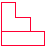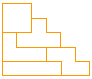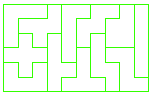Domino 2 Triominoes 5 Tetrominoes 12 Pentominoes
If we use three connected squares we find 2 different triomino shapes can be made.
If you have played Tetris then you will know that there are 5 shapes of 4 square tiles (tetrominoes) as shown here.
A puzzle I came across when I was 12 is a box of the 12 pentominoes, each piece being one of the ways of "tearing 5 connected square stamps from a perforated sheet" as George Martin puts it. They fit into a variety of shaped boxes but mine came in a 10x6 box. They can be fitted into it in 2339 different ways (ignoring rotations and horizontal and vertical flips of the box).
Puzzle Can you fit all 12 pentominoes in a box of size 12x5? 15x4? What about 3x20? [Answers].
So from domino, triomino (or triomino), tetromino, pentomino, etc we get the family of Poly-ominoes!
Puzzle How many hexominoes (6 squares) can you find? What about heptominoes (7 squares)? [Answers]
Each set has its own mathematical jigsaw puzzles, but, to me, they are far more interesting than the pictorial jigsaw puzzles in games shops. I must make a separate page on these sometime.
• A definite "must buy" is Polyominoes by Solomon Golomb and Warren Lushbaugh, a Princeton University press paperback (1996) of 198 pages. Golomb is the inventor of polyominoes and this is the revised and expanded second edition of the original of 1965 that sparked off the polyomino puzzle craze.
• Fractals, Chaos and Power Laws, M Schroeder, W H Freeman publishers, 1991. This is another fascinating book with much on self-similar sequences and patterns, Fibonacci and Phi. I have found myself dipping into this book time and time again. There is a chapter on the forbidden five-fold symmetry and its relation to the Fibonacci rabbits. (More information and you can order it online via the title-link.)

## Footnotes

The Greeks from Euclid ( around 300BC) and before knew that there were only 5 solid shapes with all sides equal, all angles equal, so that the faces are regular polygons.

### Plato

They were also mentioned by the Greek philosopher Plato (428BC-348BC). He established an Academy in Greece and the motto over the entrance was
Let no one ignorant of geometry enter here
As a philosopher, he held the view that mathematical objects "really" existed so that they are discovered by mathematicians (in the same way that new continents are discovered by explorers) rather than invented in the way that the TV or computer were invented. Plato believed that mathematics provided the best training for thinking about science and philosophy. The five regular solids are named "Platonic Solids" today after Plato.

### Euclid

The most famous ancient book on geometry was written by Euclid (pronounced "U - klid") who lived around 300 BC and worked at the Library at Alexandria in Egypt, the foremost centre of learning in the world at that time. Actually, the book was a collection of 13 volumes, called The Elements and was the collected knowledge on geometry, superbly arranged and logically presented. It was the standard mathematics text book in Europe for centuries because it trained the reader to think logically, only relying on results that could be proved logically from self-evident starting points (axioms).
Here are some axioms:
Things that are equal to the same thing are equal to each other.
The whole is greater than the part.
It is possible to draw a circle with any point as centre and with any radius.
It is possible to draw a straight line between any two points.
From these, Euclid proved theorems such as
The angles in a triangle add up to two right angles.
One of Euclid's aims in his Elements seems to be to prove that there were only 5 solid (i.e. 3-dimensional) objects with all sides equal and all angles equal, and this occupies the final (13th) book of the Elements.

The 13 books - now available in English in a 3-volume set - are classics is every sense!

This 3-volume set is cheap and has been the standard version for many years: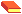The Thirteen Books of Euclid's Elements, Books 1 and 2The Thirteen Books of Euclid's Elements, Books 3 to 9The Thirteen Books of Euclid's Elements, Books 10 to 13
all are by Thomas L Heath who translated and annotated Euclid's work, each is about 464 pages, published by Dover in paperback, second edition 1956.

### Shapes for Fair Dice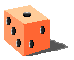We saw above that the Greeks knew of the 5 shapes that make fair dice. The Romans used a cubic dice and this is the one we most often use today.
Should we say one die or one dice?
The dictionary says that die is singular and dice is its plural form, so we ought to speak of throwing a die or two dice.
These days the plural word dice is often used for one die and the dictionary recognises this also.
A popular gambling game from at least Roman times involved throwing dice and is also called casting the dice. Some of the Roman soldiers "cast lots" for the clothes of Jesus at his crucifiction. Today we still use the phrase the die is cast. I used to think this phrase meant that a mould (US spelling=mold) had been made since we also read of someone being cast in the heroic mould as if they had been molten metal poured into a mould from which they solidify into a heroic shape. However I was wrong and it is just another use of the word die.
The real meaning of the phrase the die is cast is that a dice (one!) has been thrown (cast) meaning that, as in a game of chance, "the outcome is now fixed, the decision is made".
In these pages, I shall stick to the popular and common use, and make dice refer to the singular as well as the plural.

From the Platonic solids that we saw above, we have dice of

4 sides : the tetrahedron
6 sides: the cube (or hexahedron)
8 sides: the octahedron
12 sides: the dodecahedron
20 sides: the icosahedron
There are other shapes if we don't insist that all the sides are the same length OR we allow 2-D shapes, but which still are fair dice - i.e. each number on a face is as likely as any other number to turn up.

If we let sides be different lengths, we can have a prism which is like a new (unsharpened) pencil with flat sides.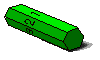Often pencils have just 6 flat sides, and we roll the pencil so that any side is likely to be face up. We can imagine a pencil with 8 sides, or 7 or even 27. If we have an odd number of sides, no one face is "up" (consider a triangular cross-sectioned pencil for instance, with just 3 choices of side). Here we may agree to use the side that the pencil lands on.

The other range of shapes is the spinner that comes with some boxed games.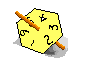Here we have a flat polygon with all sides of the same length (to make it fair). This was not in our list of Platonic solids because it is not a solid - it is just a flat 2-dimensional shape. However, we can have any number of sides and each is equally likely to be the side the spinner lands on, so it is fair.

### Bi-pyramids as dice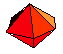Putting both of the above shapes together, we get a dice which is two n-gon-al pyramids, joined at their bases (the n-gons) to form a double pyramid or bi-pyramid. The picture shows a 12-sided dice formed from two 6-sided pyramids joined at their hexagonal bases. Perhaps we should call it a bi-hexahedral dice.

If we used pentagons then the bi-pyramidal dice would be 10-sided. It would be useful for generating random numbers up to 10.
By using two of them, say a red one for tens digits and a green one for units digits, we can roll random numbers from the hundred values between 00 and 99. If we added a blue one also, then we can get up to 999, and so on.

The advantage of the bi-pyramidal dice is that there is always a side on top no matter how the dice lands.

### Iso-hedral shapes

Here is Ed Pegg Jr.'s complete list of ALL the 3-D dice shapes which have every face identical.
It includes all our 5 Platonic solids, and, since it also includes those where not every edge is the same length, it includes the bi-pyramids too. Every face is identical to every other face, so all the faces have exactly the same polygonal shape, but some edges have different lengths to others. There are others apart from the Platonic solids and the bi-pyramids and are some pretty weird too!
The common feature is that all of them would make good dice.
Since every face is the same, they are called iso-hedral.

With thanks to Robert Popa for recommending the following:
More isohedral (i.e same-faced) dice where the edge lengths are not equal but the faces are all identical.
You can purchase some of these non-standard dice at Game shops or online, for example at Crystal Caste .© 1996-2016 Dr Ron Knott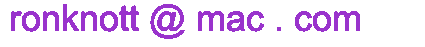updated 26 September 2016